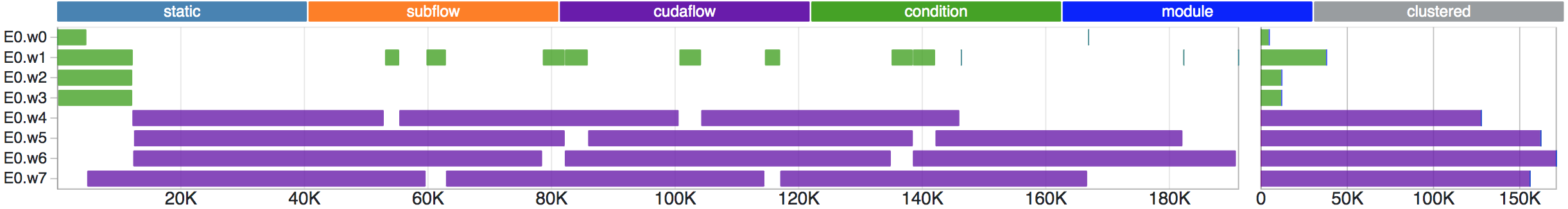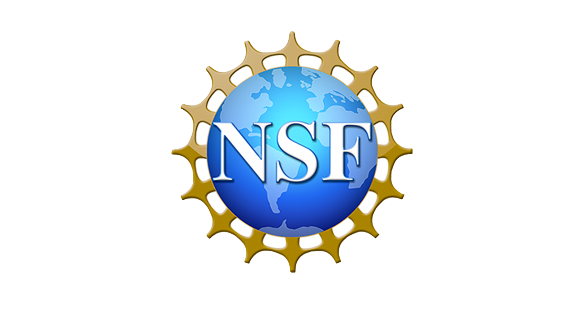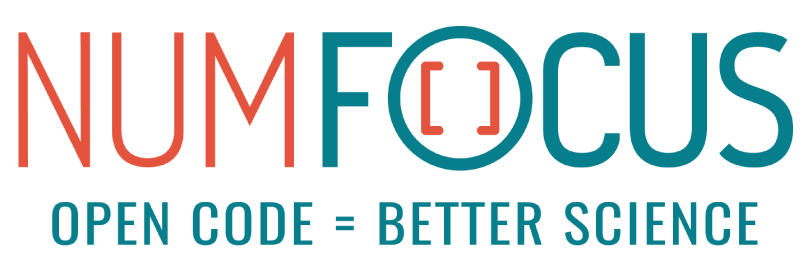# Modern C++ Parallel Task Programming

Taskflow helps you quickly write parallel and heterogeneous task programs with high performance and simultaneous high productivity. It is faster, more expressive, fewer lines of code, and easier for drop-in integration than many of existing task programming libraries. The source code is available in project GitHub.

The following program (`simple.cpp`) creates four tasks `A`, `B`, `C`, and `D`, where `A` runs before `B` and `C`, and `D` runs after `B` and `C`. When `A` finishes, `B` and `C` can run in parallel.

```#include <taskflow/taskflow.hpp>  // Taskflow is header-only

int main(){

tf::Executor executor;

auto [A, B, C, D] = taskflow.emplace(  // create four tasks
[] () { std::cout << "TaskA\n"; },
[] () { std::cout << "TaskB\n"; },
[] () { std::cout << "TaskC\n"; },
[] () { std::cout << "TaskD\n"; }
);

A.precede(B, C);  // A runs before B and C
D.succeed(B, C);  // D runs after  B and C

return 0;
}```

Taskflow is header-only and there is no wrangle with installation. To compile the program, clone the Taskflow project and tell the compiler to include the headers under `taskflow/`.

```~\$ git clone https://github.com/taskflow/taskflow.git  # clone it only once
~\$ g++ -std=c++17 simple.cpp -I taskflow -O2 -pthread -o simple
~\$ ./simple

Taskflow comes with a built-in profiler, Taskflow Profiler, for you to profile and visualize taskflow programs in an easy-to-use web-based interface.```# run the program with the environment variable TF_ENABLE_PROFILER enabled
~\$ TF_ENABLE_PROFILER=simple.json ./simple
~\$ cat simple.json
[
{"executor":"0","data":[{"worker":0,"level":0,"data":[{"span":[172,186],"name":"0_0","type":"static"},{"span":[187,189],"name":"0_1","type":"static"}]},{"worker":2,"level":0,"data":[{"span":[93,164],"name":"2_0","type":"static"},{"span":[170,179],"name":"2_1","type":"static"}]}]}
]
# paste the profiling json data to https://taskflow.github.io/tfprof/```

## Create a Subflow Graph

Taskflow supports dynamic tasking for you to create a subflow graph from the execution of a task to perform dynamic parallelism. The following program spawns a task dependency graph parented at task `B`.

```tf::Task A = taskflow.emplace([](){}).name("A");

tf::Task B = taskflow.emplace([] (tf::Subflow& subflow) { // subflow task B
tf::Task B1 = subflow.emplace([](){}).name("B1");
tf::Task B2 = subflow.emplace([](){}).name("B2");
tf::Task B3 = subflow.emplace([](){}).name("B3");
B3.succeed(B1, B2);  // B3 runs after B1 and B2
}).name("B");

A.precede(B, C);  // A runs before B and C
D.succeed(B, C);  // D runs after  B and C```

## Integrate Control Flow into a Task Graph

Taskflow supports conditional tasking for you to make rapid control-flow decisions across dependent tasks to implement cycles and conditions in an end-to-end task graph.

```tf::Task init = taskflow.emplace([](){}).name("init");

// creates a condition task that returns a random binary
tf::Task cond = taskflow.emplace([](){ return std::rand() % 2; }).name("cond");

// creates a feedback loop {0: cond, 1: stop}
init.precede(cond);
cond.precede(cond, stop);  // moves on to 'cond' on returning 0, or 'stop' on 1```

Taskflow supports GPU tasking for you to accelerate a wide range of scientific computing applications by harnessing the power of CPU-GPU collaborative computing using CUDA.

```__global__ void saxpy(int n, float a, float *x, float *y) {
int i = blockIdx.x*blockDim.x + threadIdx.x;
if (i < n) {
y[i] = a*x[i] + y[i];
}
}
tf::cudaTask h2d_x = cf.copy(dx, hx.data(), N).name("h2d_x");
tf::cudaTask h2d_y = cf.copy(dy, hy.data(), N).name("h2d_y");
tf::cudaTask d2h_x = cf.copy(hx.data(), dx, N).name("d2h_x");
tf::cudaTask d2h_y = cf.copy(hy.data(), dy, N).name("d2h_y");
tf::cudaTask saxpy = cf.kernel((N+255)/256, 256, 0, saxpy, N, 2.0f, dx, dy)
.name("saxpy");  // parameters to the saxpy kernel
saxpy.succeed(h2d_x, h2d_y)
.precede(d2h_x, d2h_y);
}).name("cudaFlow");```

## Compose Task Graphs

Taskflow is composable. You can create large parallel graphs through composition of modular and reusable blocks that are easier to optimize at an individual scope.

```tf::Taskflow f1, f2;

// create taskflow f1 of two tasks
tf::Task f1A = f1.emplace([]() { std::cout << "Task f1A\n"; }).name("f1A");
tf::Task f1B = f1.emplace([]() { std::cout << "Task f1B\n"; }).name("f1B");

// create taskflow f2 with one module task composed of f1
tf::Task f2A = f2.emplace([]() { std::cout << "Task f2A\n"; }).name("f2A");
tf::Task f2B = f2.emplace([]() { std::cout << "Task f2B\n"; }).name("f2B");
tf::Task f2C = f2.emplace([]() { std::cout << "Task f2C\n"; }).name("f2C");

.precede(f2C);```

## Launch Asynchronous Tasks

Taskflow supports asynchronous tasking. You can launch tasks asynchronously to incorporate independent, dynamic parallelism in your taskflows.

```tf::Executor executor;

// create asynchronous tasks directly from an executor
tf::Future<std::optional<int>> future = executor.async([](){
std::cout << "async task returns 1\n";
return 1;
});
executor.silent_async([](){ std::cout << "async task does not return\n"; });

// launch an asynchronous task from a running task
executor.async([](){ std::cout << "async task created within a task\n"; });
});

## Run a Taskflow through an Executor

The executor provides several thread-safe methods to run a taskflow. You can run a taskflow once, multiple times, or until a stopping criteria is met. These methods are non-blocking with a `tf::Future<void>` return to let you query the execution status.

```// runs the taskflow once
tf::Future<void> run_once = executor.run(taskflow);

// wait on this run to finish
run_once.get();

// run the taskflow four times

// runs the taskflow five times
executor.run_until(taskflow, [counter=5](){ return --counter == 0; });

// blocks the executor until all submitted taskflows complete
executor.wait_for_all();```

## Leverage Standard Parallel Algorithms

Taskflow defines algorithms for you to quickly express common parallel patterns using standard C++ syntaxes, such as parallel iterations, parallel reductions, and parallel sort.

```// standard parallel CPU algorithms
tf::Task task1 = taskflow.for_each( // assign each element to 100 in parallel
first, last, [] (auto& i) { i = 100; }
);
tf::Task task2 = taskflow.reduce(   // reduce a range of items in parallel
first, last, init, [] (auto a, auto b) { return a + b; }
);
tf::Task task3 = taskflow.sort(     // sort a range of items in parallel
first, last, [] (auto a, auto b) { return a < b; }
);

// standard parallel GPU algorithms
tf::cudaTask cuda1 = cudaflow.for_each( // assign each element to 100 on GPU
dfirst, dlast, [] __device__ (auto i) { i = 100; }
);
tf::cudaTask cuda2 = cudaflow.reduce(   // reduce a range of items on GPU
dfirst, dlast, init, [] __device__ (auto a, auto b) { return a + b; }
);
tf::cudaTask cuda3 = cudaflow.sort(     // sort a range of items on GPU
dfirst, dlast, [] __device__ (auto a, auto b) { return a < b; }
);```

Additionally, Taskflow provides composable graph building blocks for you to efficiently implement common parallel algorithms, such as parallel pipeline.

```// create a pipeline to propagate five tokens through three serial stages
tf::Pipeline pl(num_lines,
tf::Pipe{tf::PipeType::SERIAL, [](tf::Pipeflow& pf) {
if(pf.token() == 5) {
pf.stop();
}
}},
tf::Pipe{tf::PipeType::SERIAL, [](tf::Pipeflow& pf) {
printf("stage 2: input buffer[%zu] = %d\n", pf.line(), buffer[pf.line()]);
}},
tf::Pipe{tf::PipeType::SERIAL, [](tf::Pipeflow& pf) {
printf("stage 3: input buffer[%zu] = %d\n", pf.line(), buffer[pf.line()]);
}}
);

## Visualize Taskflow Graphs

You can dump a taskflow graph to a DOT format and visualize it using a number of free GraphViz tools such as GraphViz Online.

```tf::Taskflow taskflow;

A.precede(B, C, E);
C.precede(D);
B.precede(D, E);

// dump the graph to a DOT file through std::cout

## Supported Compilers

To use Taskflow, you only need a compiler that supports C++17:

• GNU C++ Compiler at least v8.4 with -std=c++17
• Clang C++ Compiler at least v6.0 with -std=c++17
• Microsoft Visual Studio at least v19.27 with /std:c++17
• AppleClang Xode Version at least v12.0 with -std=c++17
• Nvidia CUDA Toolkit and Compiler (nvcc) at least v11.1 with -std=c++17
• Intel C++ Compiler at least v19.0.1 with -std=c++17
• Intel DPC++ Clang Compiler at least v13.0.0 with -std=c++17 and SYCL20

Taskflow works on Linux, Windows, and Mac OS X.

## Get Involved